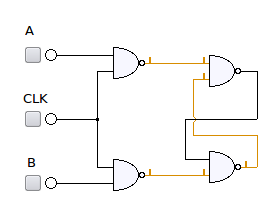- DSP log - http://www.dsplog.com -

GATE-2012 ECE Q6 (digital)

Posted By Krishna Sankar On October 7, 2012 @ 5:59 pm In GATE | 6 Comments

Question 6 on digital circuit from GATE (Graduate Aptitude Test in Engineering) 2012 Electronics and Communication Engineering paper.

Q6. Consider the given circuitIn this circuit, the race around

(A) does not occur

(B) occurs when CLK=0

(C) occurs when CLK=1 and A=B=1

(D) occurs when CLK=1 and A=B=0

Solution

Looking up the definition of Race Condition from Wiki  :

“A race condition or race hazard is a type of flaw in an electronic or software system where the output is dependent on the sequence or timing of other uncontrollable events. The term originates with the idea of two signals racing each other to influence the output first.

A typical example of a race condition may occur in a system of logic gates, where inputs vary. If a particular output depends on the state of the inputs, it may only be defined for steady-state signals. As the inputs change state, a small delay will occur before the output changes, due to the physical nature of the electronic system. For a brief period, the output may change to an unwanted state before settling back to the designed state.”

Using the truth table of NAND gates, we know that

,

, irrespective of the values of .

The circuit shown in the figure is a gated SR latch circuit using NAND gates [Ref: 2, 3].Figure : Gated SR latch using NAND gates

The state transition behavior is as follows :

Table : State transition table for gated SR-latch using NAND gates

Update (11th Oct 2012):

Given that there were some clarification raised by Mr. Raghav in the comments section, let us try to review the answers

 Choices Remark (A) does not occur Clearly, this is not true. The race around can occur when the input condition transitions from {X=Y=0} to {X=Y=1}.The {X=Y=0} is caused when {CLK=A=B=1}.The {X=Y=1} can be caused by either {CLK=0, A=x, B=x} inputs OR {CLK=1, A=B=0} input condition. (B) occurs when CLK=0 This is partially true.If the previous input condition was {CLK=A=B=1}, then changing to CLK=0 can cause race aroundNote : On power up, if CLK=0 then the system can go and settle into an indeterminate state. (C) occurs when CLK=1 and A=B=1 This is partially true. If the next input condition is either {CLK=0} or {CLK=1, A=B=0}, can cause race around (D) occurs when CLK=1 and A=B=0 This is partially true. If the previous input condition was {CLK=1, A=B=1}, then changing input to {CLK=1, A=B=0} can cause race around.

Based on the above, the lawyer in me feels that choices (B), (C), (D)  are all partially true. However as an engineer, the common factor for the cause of race around condition is {CLK=1, A=B=1}.

Given so, the right choice is :  (C) i.e. race condition can occur when CLK=1 and A=B=1 (with high probability)Note: Am looking for an easy to read text book which explains in transistor level the logic gates. Please drop a comment or send me an email.

References

 GATE Examination Question Papers [Previous Years] from Indian Institute of Technology, Madras http://gate.iitm.ac.in/gateqps/2012/ec.pdf 

 SE 271 — Introduction to Digital Systems Supplementary Reading Some Basic Memory Elements http://ecse.bd.psu.edu/cse271/memelem.pdf 

 Digital Electronics http://ptuece.loremate.com/die/node/11 

 Sequential Circuits http://www.elex.dauniv.ac.in/Kirti_Elex/PDF/Sequential_Circuit_1.pdf 

 SR Flip-Flop http://www.electronics-tutorials.ws/sequential/seq_1.html 

 Race Condition from Wiki 

URL to article: http://www.dsplog.com/2012/10/07/gate-2012-ece-q6-digital/

URLs in this post:

 Race Condition from Wiki: http://en.wikipedia.org/wiki/Race_condition

 http://gate.iitm.ac.in/gateqps/2012/ec.pdf: http://gate.iitm.ac.in/gateqps/2012/ec.pdf

 http://ecse.bd.psu.edu/cse271/memelem.pdf: http://ecse.bd.psu.edu/cse271/memelem.pdf

 http://ptuece.loremate.com/die/node/11: http://ptuece.loremate.com/die/node/11

 http://www.elex.dauniv.ac.in/Kirti_Elex/PDF/Sequential_Circuit_1.pdf: http://www.elex.dauniv.ac.in/Kirti_Elex/PDF/Sequential_Circuit_1.pdf

 http://www.electronics-tutorials.ws/sequential/seq_1.html: http://www.electronics-tutorials.ws/sequential/seq_1.html